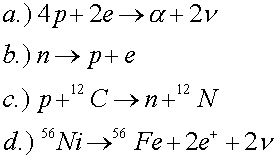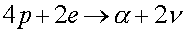Examples for nuclear physics

Example #1

Problem:

If 500 kg of nuclear matter were all in one spherical drop, what would the radius of the drop be?

Solution:

First figure out the number of particles by dividing by the mass of the proton, 1.67 E-27 kg. This gives, that the number of nucleons is A = 2.99 E29. Next use the formula, R = A1/3 x1.2 E-15 m.

R = 8.02 E-6 m

Example #2

Problem:

Which of the following reactions violate conservation of baryon number, electric charge or lepton number?Solution:

The second reaction violates lepton number. The last two reactions are allowed, but one must know (see periodic table) that carbon has a charge of 6, nitrogen has a charge of 7, nickel has a charge of 28 and iron (Fe) has a charge of 26.

b

Example #3

Problem:

a.) What energy (in MeV) is released by the reaction,?

DATA: mp = 1.007276 u,   ma = 4.001506 u,    me = 0.000549 u

Solution:

Neutrinos are massless, so they just carry energy. One then calculates the difference of the proton mass (times 4) plus twice the electron mass minus the alpha mass. Then multiply by 931.5 to get the energy in MeV.

Q = 26.7 MeV

b.) If one kilogram of protons and electrons were converted into alphas, how many joules of energy would be generated?

Solution:

This can be done several ways. The number of reactions will be one fourth of Avogadro's number, NA = 6.02 E26. Multiplying by the energy per reaction, and then converting to Joules by multiplying by 1.602 E-13 gives

6.44 E4 J

Nuclear physics' index